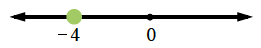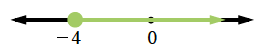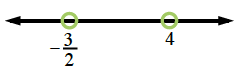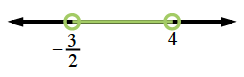### Home > CCA2 > Chapter Ch4 > Lesson 4.2.1 > Problem4-65

4-65.

Find boundary points for each of the following inequalities. Draw the boundaries on a number line and shade the solution regions.

1. $3x + 2 ≥ x − 6$

Solve for $x$ to find the boundary point(s).

$3x+2=x−6\\2x=−8\\x=−4$

Since $x=−4$, plot $−4$ on a number line.
Since $−4$ makes the inequality true, shade the point.Test a point on either side of the boundary point in the original inequality and decide if you get a true or false statement.

If you choose $x=0$:
$3(0)+2\ge(0)−6$
$2\ge−6→ \text{True}$

If you choose $x=−6$:
$3(−6)+2\ge(−6)−6$
$−16\ge−12→$ False

Shade the points that are on the true side, to the right of $−4$.1. $2x^2 − 5x < 12$

Solve for $x$ to find the boundary point(s).

$2x^2−5x=12\\2x^2−5x−12=0$

Plot the boundary points on a number line. Since the points do not make the inequality true, do not shade them.Test a point between the boundary points in the original inequality. Decide if the simplified statement is true or false.

If you choose $x=0$:
$2(0)^2−5(0)<12$
$0<12→ \text{True}$

Shade the points between the boundary points, the true region.